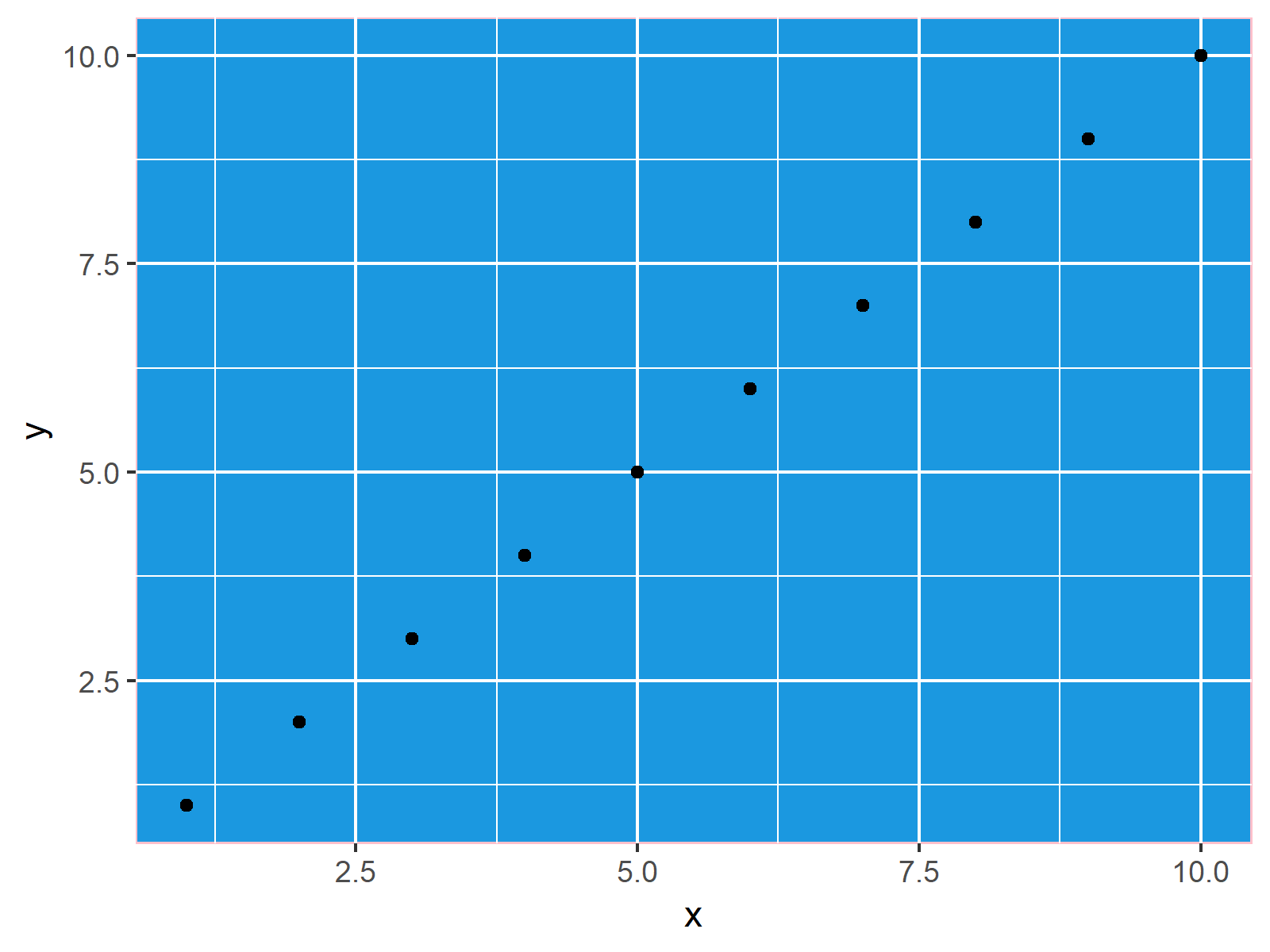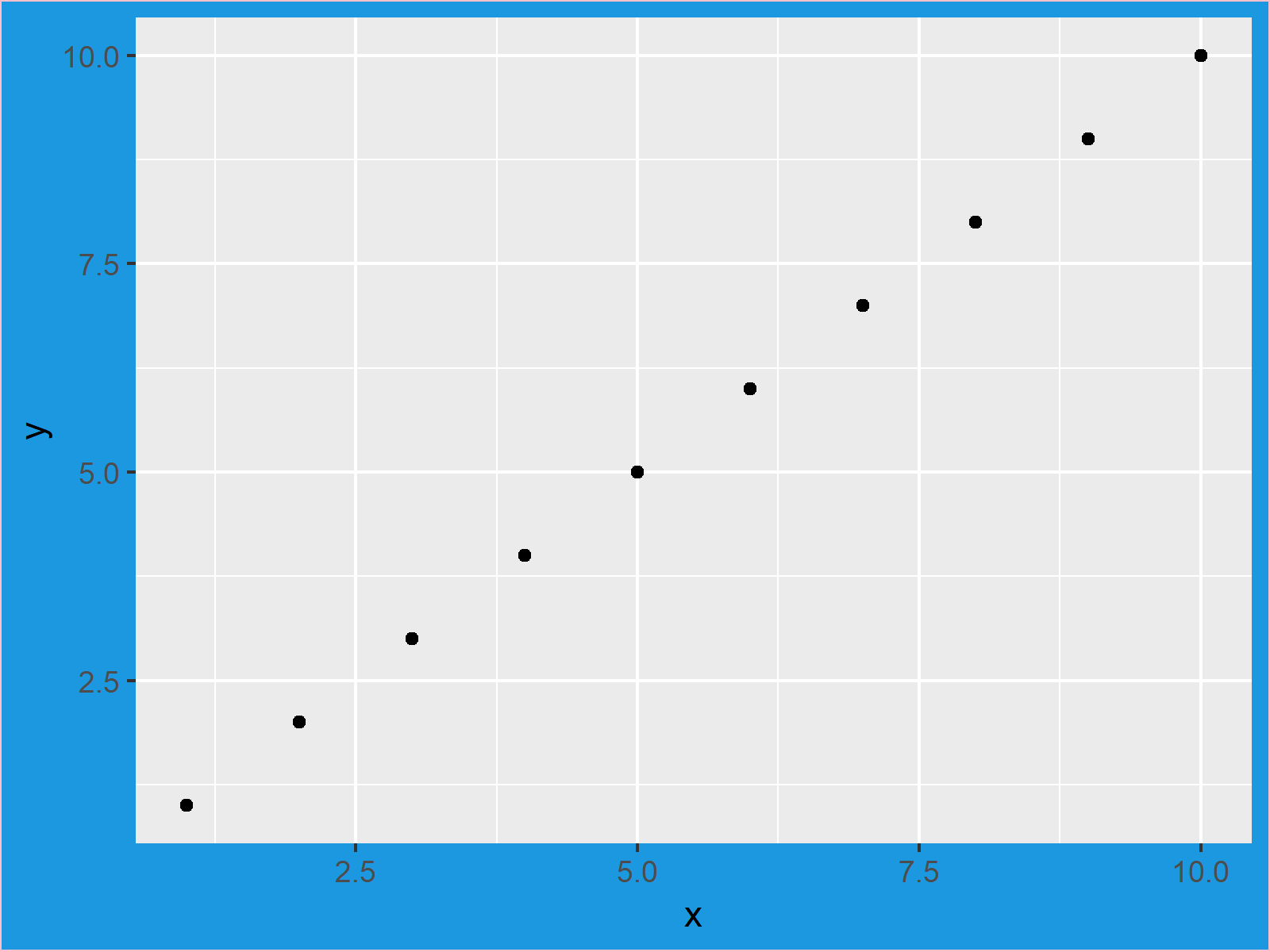# Change Background Color of ggplot2 Plot in R (3 Examples)

In this article you’ll learn how to modify background colors of ggplot2 plots in the R programming language.

The article consists of this content:

Let’s get started!

## Creation of Example Data

I’ll use the following example data frame for the examples of this R tutorial. Our data contains ten rows and two numeric columns with values ranging from 1 to 10:

```data <- data.frame(x = 1:10,             # Create example data
y = 1:10)```

Furthermore, we have to install and load the ggplot2 package to R:

```install.packages("ggplot2")              # Install & load ggplot2
library("ggplot2")```

Now, we can draw a simple ggplot2 graph as follows:

```ggp <- ggplot(data, aes(x, y)) +         # Create basic ggplot
geom_point()
ggp                                      # Draw plot in RStudio```Figure 1: Default Colors of ggplot2 Package.

Our example plot is shown in Figure 1. It’s a basic scatterplot with default specifications of the background colors.

## Example 1: Changing Panel Background Color of ggplot2 Plot

The background of a ggplot2 graphic consists of different parts. In Example 1, you’ll learn how to use the theme function and the panel.background argument to change the panel background of a ggplot2 plot. Have a look at the following R code:

```ggp +                                    # Change panel background
theme(panel.background = element_rect(fill = "#1b98e0", color = "pink"))```Figure 2: Different Colors of Panel Background.

Figure 2 shows the output of the previous R syntax. As you can see, the previously grey areas are now colored blue (i.e. hex color code #1b98e0) and the line around the panel background is pink.

## Example 2: Changing Plot Background Color of ggplot2 Plot

Example 2 shows how to modify the plot background of a ggplot2 graph, i.e. the white area around the plot. Consider the R code below:

```ggp +                                    # Change plot background
theme(plot.background = element_rect(fill = "#1b98e0", color = "pink"))```Figure 3: Different Colors of Plot Background.

Figure 3 illustrates the output of our previous code. The panel is shown in the default grey/white grid and the background of the plot is shown in blue and pink.

## Example 3: Changing Theme of ggplot2 Plot

As you have seen in Examples 1 and 2, you can manually replace the colors of the different parts of a ggplot. However, it is also possible to switch the entire ggplot2 theme. For instance, you may use the theme_bw instead of the default ggplot2 theme:

```ggp +                                    # Change ggplot2 theme
theme_bw()```Figure 4: theme_bw of ggplot2 Package.

Figure 4 shows how our plot looks like when selecting theme_bw.

Note that the previous examples can be applied to any type of ggplot2 plot (i.e. bar charts, line plots, histograms, boxplots, and so on…).

## Video, Further Resources & Summary

Some time ago I have published a video on my YouTube channel, which explains the R code of this tutorial. You can find the video below:

Please accept YouTube cookies to play this video. By accepting you will be accessing content from YouTube, a service provided by an external third party.If you accept this notice, your choice will be saved and the page will refresh.

Furthermore, you may want to read some of the other tutorials of this website. Please find a selection of articles below.

In summary: In this R tutorial you learned how to improve background colors and grids made with the ggplot2 package. If you have additional questions, don’t hesitate to let me know in the comments section.

Subscribe to the Statistics Globe Newsletter